MATLAB Function Referencegradient

Numerical gradient

Syntax

• ```FX = gradient(F)
[FX,FY] = gradient(F)
[Fx,Fy,Fz,...] = gradient(F)
[...] = gradient(F,h)
[...] = gradient(F,h1,h2,...)
```

Definition

The gradient of a function of two variables,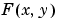, is defined as

•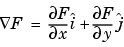and can be thought of as a collection of vectors pointing in the direction of increasing values of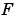. In MATLAB, numerical gradients (differences) can be computed for functions with any number of variables. For a function of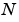variables,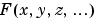,

•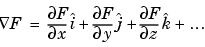Description

```FX = gradient(F) ``` where `F` is a vector returns the one-dimensional numerical gradient of `F`. `FX` corresponds to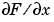, the differences in the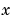direction.

```[FX,FY] = gradient(F) ``` where `F` is a matrix returns theand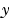components of the two-dimensional numerical gradient. `FX` corresponds to, the differences in the(column) direction. `FY` corresponds to, the differences in the(row) direction. The spacing between points in each direction is assumed to be one.

```[FX,FY,FZ,...] = gradient(F) ``` where `F` has `N dimensions` returns the `N` components of the gradient of `F`. There are two ways to control the spacing between values in `F`:

• A single spacing value, `h`, specifies the spacing between points in every direction.
• `N` spacing values (`h1,h2,...`) specifies the spacing for each dimension of `F`. Scalar spacing parameters specify a constant spacing for each dimension. Vector parameters specify the coordinates of the values along corresponding dimensions of `F`. In this case, the length of the vector must match the size of the corresponding dimension.

```[...] = gradient(F,h) ``` where `h` is a scalar uses `h` as the spacing between points in each direction.

```[...] = gradient(F,h1,h2,...) ``` with `N` spacing parameters specifies the spacing for each dimension of `F`.

Examples

The statements

• ```v = -2:0.2:2;
[x,y] = meshgrid(v);
z = x .* exp(-x.^2 - y.^2);
[px,py] = gradient(z,.2,.2);
contour(v,v,z), hold on, quiver(v,v,px,py), hold off
```

produce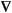Given,

• ```F(:,:,1) = magic(3); F(:,:,2) = pascal(3);
```gradient(F)
``````

takes `dx` = `dy` = `dz` = `1`.

• ````[PX,PY,PZ] = gradient(F,0.2,0.1,0.2)`
```

takes `dx = 0.2`, `dy = 0.1`, and `dz = 0.2`.

See Also

`del2`, `diff`

© 1994-2005 The MathWorks, Inc.